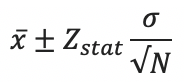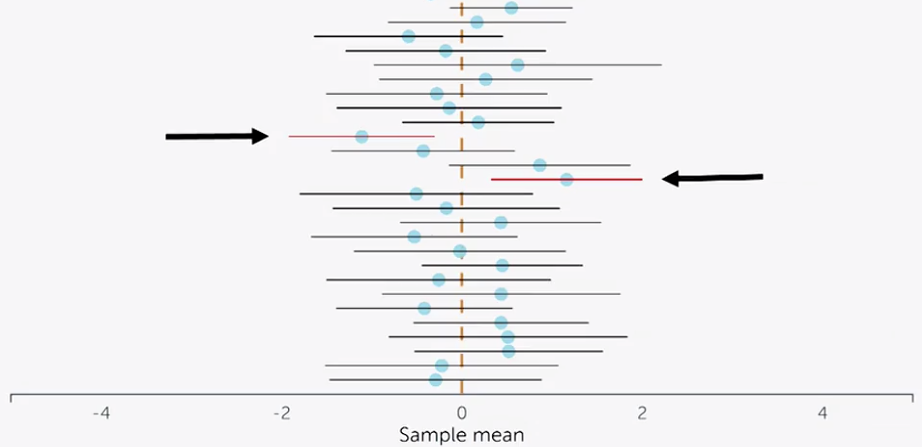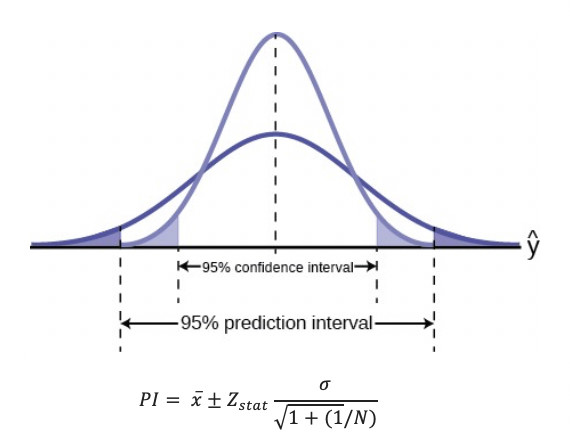# P-values and Confidence Intervals

## Ro Data Sip-and-Share Q1 2019

Getting a firm understanding of key statistical concepts is critical in interpreting and sharing results from various analyses. Using Daniel Laken’s Improving your statistical inferences course, I set out to get precise definitions of two widely used but commonly misconstrued concepts: p-values and confidence intervals.

#### P-values

A p-value is the probability the getting the observed (or more extreme) result of a test if the null hypothesis (H0) is true. The null hypothesis for a test is that there is no difference between two groups. Stated alternatively, a p-value is the chance of getting the observed result if the two groups you were testing were in fact exactly the same.

For example, imagine that you have a coin which you suspect is a biased coin that is weighted towards heads. The null hypothesis is that this is a fair coin, and your alternative hypothesis is that it is weighted towards heads.

You flip the coin 10 times, and get 8 heads and 2 tails.

Using the binomial distribution formula (since there are only two outcomes to this test), we find that the p-value for this test is 0.055. This is the probability of getting 8, 9, or 10 heads from 10 flips. If you would like a further explanation in using this formula, I found this video quite helpful.

When you interpret the p-value from this test, you do not want to make a statement about whether your hypothesis is true or not. You want to make a statement about the data, because that’s what the p-value relates to.

So, the p-value won’t tell you whether the coin is fair or not, but it will tell you the probability that you’d get at least that many heads if the coin was fair.

In this example, if the coin was actually fair, there would be a 5.5% chance that you’d get 8 or more heads when you flipped it 10 times.

Let’s redo the example but say that out of 10 tosses you observed 6 heads. In this case, the p-value from this test is 0.377. Putting the p-value into English, we are saying that if the coin is fair there is a 37.7% chance that out of 10 tosses, we would get 6 or more heads. Why is this so much higher than the 5.5% from the previous example? In intuitive terms, 10 is really not a lot of tosses — it’s quite plausible that a perfectly fair coin could give you 6 heads and 4 tails instead of 5 each. You’d need a lot more tosses (i.e. a much larger sample size) to be sure that the coin really is biased towards giving heads 60% of the time.

#### Confidence Intervals

When talking about statistics, it is important to distinguish between studying a sample and studying a population. We very rarely have the ability to run tests against the whole population, so we take samples of the population to run our tests on.

Now, a sample is only part of the population, so parameters (summary numbers such as the mean, or standard deviation) will differ between the sample and the population.

Confidence intervals help us approximate the true population parameters given a sample parameter estimate. A confidence interval has a lower bound and an upper bound to account for the fact that the true mean is not known but that we can guess at it based on the sample mean.

Take the example of measuring average height. If we measure 100 people and get an average height of 6'1", that average height is only a sample mean. If we wanted to use this sample data to come up with an interval of heights within which we were 95% sure that the true population mean would lie (i.e. the 95% confidence interval), that interval might look like 5'4" — 6'10" (i.e. 6'1" +/- 9").

For normally distributed data, confidence intervals are calculated using the sample mean, the Z statistic for the confidence level chosen (1.96 for a 95% confidence interval), the sample standard deviation, and the size of the sample population.Confidence Interval Formula

The Z statistic for a given confidence level can be found using a z-table. A higher confidence level or standard deviation will give a wider confidence interval, while a larger sample population will give a narrower confidence interval.[Source: Interpreting Confidence Intervals via rpsychologist]

The visualization shows many different confidence intervals from many tests within the same study. The horizontal lines are the confidence intervals, the blue dots are the sample estimate means, and the red dashed vertical line is the true population mean (in this case it is zero). For 95% confidence intervals, you can easily see that most of the confidence intervals pass through the true population mean, but approximately 5% of the time, a confidence interval will not contain the true population mean (these instances are marked in red).

Confidence intervals are also sometimes confused with prediction intervals. Prediction intervals give you a range of values where you can expect to see the next data point based on an existing model. The key difference is that a prediction interval tells you about a predicted future observation, while a confidence interval tells you about the likely distribution of the true population parameter (in our case, the mean). For normally distributed data, the formula for a prediction interval is nearly identical to the formula used for a confidence interval, but with an added error term. There is greater uncertainty when you predict a single data point, so prediction intervals are always wider than confidence intervals.Source: Introductory Business Statistics via CNX

It is important to remember that a single p-value or confidence interval by itself should not be used to prove or disprove a hypothesis, but rather it should be seen as an invitation to explore an effect further.